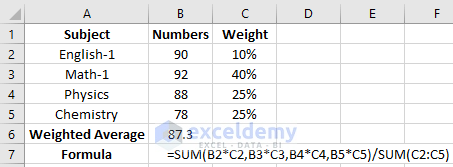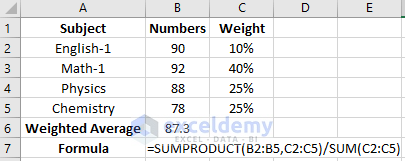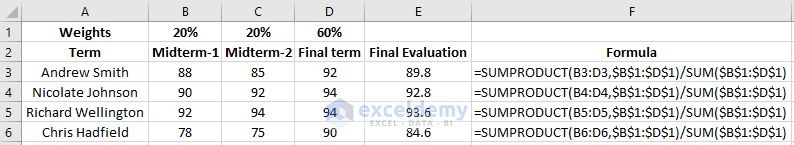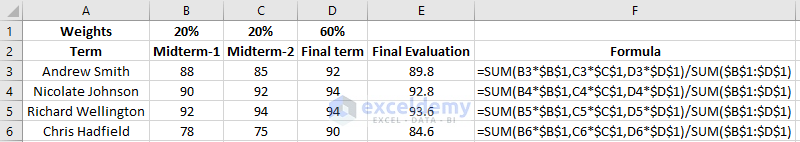Disclosure: This post may contain affiliate links, meaning when you click the links and make a purchase, we receive a commission.

# How to calculate weighted average in Excel with percentages (2 ways)

In this article, I will show you how to calculate the weighted average in Excel with percentages. The weighted average is the average where some numbers are taken into consideration as the weighted elements for finding the average. It is different from the normal average as the weighted elements contribute to the final result more heavily than the other elements. Here I will calculate the weighted average with percentages.

## Calculating Weighted Average with Percentage

Let`s say you achieved different numbers in different subjects. You need to carry out the weighted average where different weights are given in different subjects. It can be calculated in two ways. Let’s look into the below picture where one process is done.Here the formula which has been used here is, =SUM(B2*C2,B3*C3,B4*C4,B5*C5)/SUM(C2:C5). Drag this formula for the rest of the cells of the column to get the result for the whole column.

The other way of calculating the weighted average is using the SUMPRODUCT function with the SUM function as a formula.Here the formula that has been used here is, =SUMPRODUCT(B2:B5,C2:C5)/SUM(C2:C5). Drag this formula for the rest of the cells of the column to get the result for the whole column.

## Weighted Average Calculation for Multiple Terms

Suppose there is a list of students who achieved different marks in a single subject in different terms. Each term consists of different percentage weight. Considering the percentage weight, you need to calculate the final marks for each of the students. Here we will be using the same formula. But instead of using the arrays column, we will use the row column. Look into the below example for getting a clear view.Here the formula that is used in this calculation is, =SUMPRODUCT(B3:D3,\$B\$1:\$D\$1)/SUM(\$B\$1:\$D\$1). Drag this formula for the rest of the cells of the column to get the result for the whole column. You can use the SUM function instead of the SUMPRODUCT function which will give you the same result. In that case, the formula will be, =SUM(B3*\$B\$1,C3*\$C\$1,D3*\$D\$1)/SUM(\$B\$1:\$D\$1)Note: In this case cell B1, C1, D1 is taken as the absolute reference because they will be used in the same manner for each of the cells of the Final Evaluation column.

How to Calculate Weighted Average in Excel with Percentages

## Conclusion

As you can see, you can calculate the weighted average in Excel with percentages using two formulas. You can use any of the two formulas which will give you the same result. This calculation might be useful for calculating grades and numbers for the students. Also, you can apply these in many statistical analyses.

Hello!

Welcome to my Profile. Here I will be posting articles related to Microsoft Excel. I have completed my BSc in Electrical and Electronic Engineering from American International University-Bangladesh. I am a diligent, goal-oriented engineer with an immense thirst for knowledge and attitude to grow continuously. Continuous improvement and life-long learning is my motto.

1 Comment
1. ReplyKim Aug 5, 2018 at 10:03 PM

Thank you so much this was insightful looking forward to more articles and info

Much appreciates